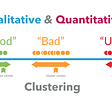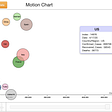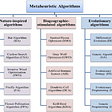# The Description from Kaggle(Data Resource)

• Listings, including full descriptions and average review score
• Reviews, including unique id for each reviewer and detailed comments
• Calendar, including listing id and the price and availability for that day

# Question 1: What’s the relation between position and price?

`# this step is for variable definition and convert the 'price' column to float(It was object initially)# Also, because the price has dollar sign and decimal point, I'll remove it in this block of code.king_station_position = [47.598330, -122.311640]latitude = []longtitude = []distance = []listing['price'] = (listing['price'].replace('[\\$,)]', '', regex=True).replace('[(]', '-', regex=True)).astype(float)`
`# We're using the price column and there's no NaN value. - No need to data cleaning.# remove outlier, mean +/- 3 stddelete = []for i in range(len(listing['price'])):    if listing['price'].iloc[i] > (listing['price'].mean() + 3 * listing['price'].std()) or listing['price'].iloc[i] < (listing['price'].mean() - 3 * listing['price'].std()):        delete.append(listing['price'].index[i])listing = listing.drop(delete)`
`128 + 90 * 3 = 398`
`# the distance between the airbnb's position and the stationfor index in range(len(listing)):    latitude.append(abs(king_station_position - listing['latitude'].iloc[index]))    longtitude.append(abs(king_station_position - listing['longitude'].iloc[index]))             # sqrt(latitude^2 + longtitude ^ 2) = linear distancefor index in range(len(latitude)):    distance.append(math.sqrt(latitude[index] ** 2 + longtitude[index] ** 2))`
`# visualizing# price / accomodates means price per personplt.scatter((listing['price'] / listing['accommodates']), distance, s=3)plt.xlabel("Price")plt.ylabel("Relative Distance")plt.title("Relation between price and distance");`

# Q2: What about the relationship between the amounts of review and price? Are they positive correlation?

`# the amount of review, sorting by listing_id. Type is seriesamount_of_reviews = reviews.listing_id.value_counts().sort_index()amount_of_reviews.head()`
`# sort the 'listing' by id, reset its index and drop the initial indexlisting = listing.sort_values('id').reset_index(drop=True)listing.head()`
`# Convert 'amount_of_reviews' to dataframe for merging, and reset its index# After reseting its index, renaming its columns nameamt_reviews_df = amount_of_reviews.to_frame().reset_index()amt_reviews_df = amt_reviews_df.rename(columns={'index': 'id', 'listing_id': 'review_amounts'})amt_reviews_df.head()`
`# merge 'amt_reviews_df' and 'listing' on 'id'# For some airbnb without reviews, use left method.listing = listing.merge(amt_reviews_df, on='id', how='left')listing.loc[:, ['id', 'accommodates', 'price', 'review_amounts']]`
`# visualizing# price / accmmodates means price per person(Am I right? I'm not sure.)plt.figure(figsize=(20, 10))plt.scatter(x=(listing['price'] / listing['accommodates']),             y=listing['review_amounts'],             s=15)plt.xlabel("Price")plt.ylabel("The amount of reviews")plt.title("Relation between price and reviews");`

# Q3: Does it have busy season? If have, is it more expensive than usual?

1. convert the ‘available’ columns’ t/f to 0/1
2. calculate the total of a day of availability by adding all airbnbs’ data
3. visualizing it
`# convert the 'available' columns' t/f to 0/1available_mapping = {'t': 0, 'f': 1}calendar['available'] = calendar['available'].map(available_mapping)calendar`
`# here we change the data type from object to datatime.# Also we calculate the mean on everyday. It can show us the availability.calendar['date'] = pd.to_datetime(calendar['date'])availability = calendar.set_index('date').groupby(pd.Grouper(freq='d')).mean()availability = availability.reset_index()availability`
`# Then we plotplt.figure(figsize=(20, 10))plt.plot(availibility['date'], availibility['available'])plt.show()`
`# here we're going to convert the price to integercalendar.price = calendar.price.fillna('\$0.00')calendar.price = calendar['price'].replace('[\\$,)]','', regex=True).replace('[(]','-', regex=True).astype(float)calendar.price`
`price_avg = calendar.set_index('date').groupby(pd.Grouper(freq='d')).sum()price_avg = price_avg.reset_index()price_avg`

--

--

--

## More from Joe Chao

Love podcasts or audiobooks? Learn on the go with our new app.

## Hello world## Mapping Qualitative Reasoning to Quantitative Analytics## Covid-19: Genetic Mutation or Engineering## How to present Covid-19 information in the United States? Design for Understanding## Metaheuristic algorithms## The Perils of Palette Transfer## Experimenting with digital tools to engage, collect, and share information in times of COVID-19## Top 3 projects with the highest ROI index## Preppin Data: 2020 week 1## Digital Craft FINALS: Fullstack Capstone## “Summary of 2021 “ from Storange Cloud